# Introduction

So far, we have explained how to set up the RAPIDS functionality to use GPU for machine learning. In this article, we will walk you through the actual power of utilizing RAPIDS in comparison to Sklearn, along with their performance assessment with the classification use case. But before diving in, let’s clear out some things that you have to know about the use case.

# About the Use Case & Data

The use case is the loan default prediction and you can download it directly from here. The dataset is about the customers being defaulters ranging from the year 2007 to 2015.

To load the data, we will use be using the pandas read_csv() method.

`path = "https://gitlab.com/mkdsr09/industry-analytics/-/raw/main/Finance/02%20Analysis%20&%20Detection%20of%20Credit%20Default%20Risk/LoanDefaultData.csv"data = pd.read_csv(filepath_or_buffer=path)print(‘Data Shape:’, data.shape)Output:Data Shape: (887379, 22)`

# Data Cleaning

Next, we will clean/handle the data to prepare it for our model development. Please note that we have already identified the steps that will take place to clean the data. You may go ahead and analyze the data before taking any action.

`# Pre Identified: No null values present, No duplicates present# Dropping unnecessry featuresdata.drop(['cust_id', 'date_issued', 'date_final', 'state'], axis = 1, inplace=True)`

# Feature Encoding

In this step, we will be encoding all the categorical features to numeric and then proceed with data scaling. Here we will proceed with two popular methods of encoding categorical features i.e. Label Encoding and Dummy Encoding (One hot encoding).

`# Label Encodingordered_labels = ['year', 'income_type', 'app_type', 'interest_payments', 'grade', 'loan_duration']encode = LabelEncoder()for i in ordered_labels:    if isinstance(data[i].dtype, object):        data[i] = encode.fit_transform(data[i])# One hot encodingdata = pd.get_dummies(data=data, columns=['own_type', 'loan_purpose'])print('Label Encoding Success!')print('Data Shape:', data.shape)Output:Label Encoding Success! Data Shape: (887379, 36)`

# Feature Scaling (Standardization)

In this step, we will scale our data and split the data into input and target format so that we can proceed with the feature selection part.

`X, y = data.drop(columns='is_default', axis=1), data['is_default']print('X & y Shape:', X.shape, y.shape)# Feature Scaling (Standardization)std_scale = StandardScaler()scale_fit = std_scale.fit_transform(X)X_data = pd.DataFrame(scale_fit, columns = X.columns)print('Data Shape:', X_data.shape)Output:X & y Shape: (887379, 35) (887379,)Data Shape: (887379, 35)`

# Feature Selection

Now that we have all the features on one scale, we will use an algorithmic approach to find essential data features for model development. In this step, we will be using a random forest model to get a subset of features (best) for model development.

`# Have some patience, may take some time :)sel = SelectFromModel(RandomForestClassifier(n_estimators=100, random_state=42, class_weight='balanced', n_jobs=-1))sel.fit(X_data, y)selected_feat= X_data.columns[(sel.get_support())].tolist()print('Total Features Selected are', len(selected_feat))# Estimated by taking mean(default) of feature importanceprint('Threshold set by Model:', np.round(sel.threshold_, decimals = 2))print('Features:', selected_feat)Output:Total Features Selected are 10 Threshold set by Model: 0.03 Features: ['year', 'emp_duration', 'annual_pay', 'loan_amount', 'interest_rate', 'dti', 'total_pymnt', 'total_rec_prncp', 'recoveries', 'installment']`

# Data Splitting

Now, we will take only those features into account which are identified as best for model development by the random forest.

`imp_feature = X_data[selected_feat]X_train, X_test, y_train, y_test = train_test_split(imp_feature, y, test_size=0.2, random_state=42, stratify=y)print('Training Data Shape:', X_train.shape, y_train.shape)print('Testing Data Shape:', X_test.shape, y_test.shape)Output:Training Data Shape: (709903, 10) (709903,) Testing Data Shape: (177476, 10) (177476,)`

# Model Development

In this step, we will develop machine models using Sklearn and RAPIDS and train them using GPUs. Please make sure that GPUs are running in your environment.

Model Development using Sklearn Algorithms

`sk_results = list()models = [skLogisticRegression(class_weight='balanced', random_state=42),          skLogisticRegression(penalty='l1', solver='liblinear', class_weight='balanced', random_state=42),          skRandomForestClassifier(class_weight='balanced', random_state=42),          skKNeighborsClassifier()]for model in models:    start_time = time.time()    model.fit(X_train, y_train)    duration = time.time() - start_time    y_train_pred = model.predict(X_train)    y_test_pred = model.predict(X_test)    accuracy = np.round(a=accuracy_score(y_train, y_train_pred), decimals=2)    train_preicision = np.round(a=precision_score(y_train, y_train_pred), decimals=2)    test_precision = np.round(a=precision_score(y_test, y_test_pred), decimals=2)    train_recall = np.round(a=recall_score(y_train, y_train_pred), decimals=2)    test_recall = np.round(a=recall_score(y_test, y_test_pred), decimals=2)    record = [type(model).__name__, duration, accuracy, train_preicision, test_precision, train_recall, test_recall]    sk_results.append(record)    print(type(model).__name__, 'Finished Training!')# Consolidate the model resultssk_results = 'LogisticRegression (L2)'sk_results = 'LogisticRegression (L1)'columns = ['Model (Sklearn)', 'Execution Time', 'Accuracy', 'Precision (Train)', 'Precision (Test)', 'Recall (Train)', 'Recall (Test)']sk_frame = pd.DataFrame(data=sk_results, columns=columns)sk_frame.to_csv('SkResults.csv', index=False)`

Model Development using RAPIDS Algorithms

`cu_results = list()models = [cuLogisticRegression(penalty='l2'),          cuLogisticRegression(penalty='l1'),          cuRandomForestClassifier(random_state=42),          cuKNeighborsClassifier()]for model in models:    start_time = time.time()    model.fit(X_train, y_train)    duration = time.time() - start_time    accuracy = np.round(a=accuracy_score(y_train, y_train_pred), decimals=2)    train_preicision = np.round(a=precision_score(y_train, y_train_pred), decimals=2)    test_precision = np.round(a=precision_score(y_test, y_test_pred), decimals=2)    train_recall = np.round(a=recall_score(y_train, y_train_pred), decimals=2)    test_recall = np.round(a=recall_score(y_test, y_test_pred), decimals=2)    record = [type(model).__name__, duration, accuracy, train_preicision, test_precision, train_recall, test_recall]    cu_results.append(record)    print(type(model).__name__, 'Finished Training!')# Consolidate the model resultscu_results = 'LogisticRegression (L2)'cu_results = 'LogisticRegression (L1)'columns = ['Model (RAPIDS)', 'Execution Time', 'Accuracy', 'Precision (Train)', 'Precision (Test)', 'Recall (Train)', 'Recall (Test)']cu_frame = pd.DataFrame(data=cu_results, columns=columns)cu_frame.to_csv('cuResults.csv', index=False)`

# Performance Analysis

Now that we have developed models using both Sklearn and RAPIDS libraries, it’s time to visualize their execution performance using the help of Plotly go. We have shown the performance using all the algorithms vs excluding random forest and Logistic Regression (L1) because it will be hard to visualize the clear difference. But before that, we need to create data frames of the results.

`sk_exclude_frame = sk_frame[(sk_frame['Model (Sklearn)'] != 'RandomForestClassifier') & (sk_frame['Model (Sklearn)'] != 'LogisticRegression (L1)')]cu_exclude_frame = cu_frame[(cu_frame['Model (RAPIDS)'] != 'RandomForestClassifier') & (cu_frame['Model (RAPIDS)'] != 'LogisticRegression (L1)')]`

Let’s plot the graph.

`fig = go.Figure()fig = make_subplots(rows=2, cols=1, subplot_titles=['Execution Performance (All Models)','Execution Performance (Excluding Random Forest & Logistic Regression (L1))'])fig.add_trace(go.Scatter(x=sk_frame['Model (Sklearn)'],y=sk_frame['Execution Time']*1000,mode='lines+markers',name='Sklearn'), row=1, col=1)fig.add_trace(go.Scatter(x=cu_frame['Model (RAPIDS)'],y=cu_frame['Execution Time']*1000,mode='lines+markers',name='RAPIDS'), row=1, col=1)fig.add_trace(go.Scatter(x=sk_exclude_frame['Model (Sklearn)'],y=sk_exclude_frame['Execution Time']*1000,mode='lines+markers',name='Sklearn'), row=2, col=1)fig.add_trace(go.Scatter(x=cu_exclude_frame['Model (RAPIDS)'],y=cu_exclude_frame['Execution Time']*1000,mode='lines+markers',name='RAPIDS'), row=2, col=1)fig.update_layout(height=600, width=1200)fig['layout']['xaxis']['title'] = 'Models'fig['layout']['xaxis2']['title'] = 'Models'fig['layout']['yaxis']['title'] = 'Execution Time (in milliseconds)'fig['layout']['yaxis2']['title'] = 'Execution Time (in milliseconds)'fig.show()`

And that’s it. We can see that RAPIDS is better than using Sklearn algorithms when it comes to utilizing GPUs.

--

--

--

One of India’s leading institutions providing world-class Data Science & AI programs for working professionals with a mission to groom Data leaders of tomorrow!

Love podcasts or audiobooks? Learn on the go with our new app.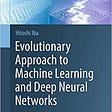## Are you ready for a Video Classification Challenge?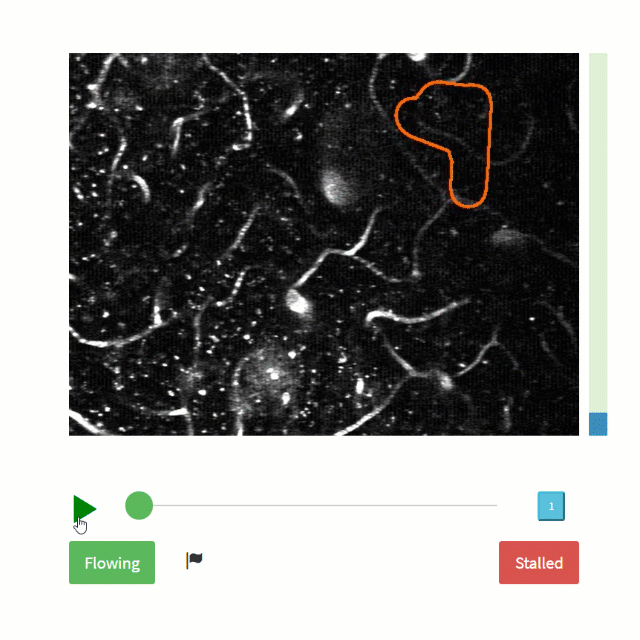## Neuromorphic computing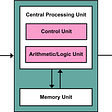## Experience Of Google Auto ML — Cloud NLP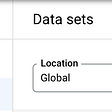## Predict or Classify — Machine Learning Approaches to Stock Selection.## Create a SMS spam classifier in python## Time series Forecasting: Using a LSTM Neural Network to predict Bitcoin prices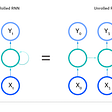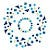## INSAID

One of India’s leading institutions providing world-class Data Science & AI programs for working professionals with a mission to groom Data leaders of tomorrow!

## Hyperparameter tuning/Tracking ML models using MLFLOW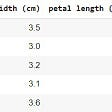## Building a Reproducible Model Workflow — ML Pipeline — Heart Failure Prediction## Using Elasticsearch for anomaly detection in time series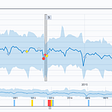## Real-time sentiment analysis using an LSTM network in TensorFlow